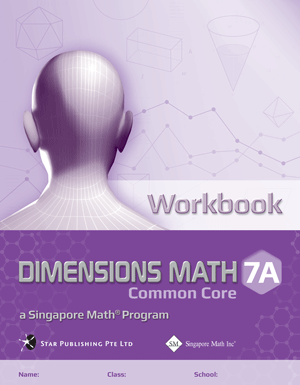# Singapore Math Dimensions Math Workbook 7A

Workbooks are the essential supplements to textbooks as they give students the opportunity to practice applying new concepts. Workbook exercises polish analytical skills and help students develop a stronger foundation in math through repetition and variation.

Features & Components:

• Basic Practice: Simple questions that drill comprehension of concepts.
• Further Practice: More difficult questions that involve direct application.
• Challenging Practice: Questions that require synthesis.
• Enrichment: Questions that demand higher order thinking, analysis, and reasoning.

The answer key at the back of the book provides answers only. Workbook Solutions (sold separately) contain fully worked solutions.

Note: Two workbooks (A and B) correspond to the two halves of the school year. Soft cover.

Dimensions Math® Textbook 7A

Chapter 1: Factors and Multiples

1.1 Factors and Multiples

A. Factors
B. Multiples

1.2 Prime Factorization and Exponential Notation

A. Prime Factorization
B. Exponential Notation

1.3 Greatest Common Factor (GCF)
1.4 Least Common Multiple (LCM)
1.5 Square Roots and Cube Roots

A. Square Roots
B. Cube Roots

In A Nutshell

Chapter 2: Real Numbers

2.1 Idea of Negative Numbers and the Number Line

A. Negative Numbers
B. The Number Line
C. Absolute Value

2.3 Subtraction and Absolute Value of the Difference

A. Subtraction of Integers
B. Absolute Value of the Difference

2.4 Multiplication, Division, and Combined Operations of Integers

A. Multiplication of Integers
B. Division of Integers
C. Combined Operations of Integers

2.5 Rational Numbers

A. Definition
B. Addition and Subtraction of Rational Numbers
C. Multiplication and Division of Rational Numbers

2.6 Real Numbers and Use of Calculators

A. Real Numbers
B. Use of Calculators

2.7 Rounding Numbers to Decimal Places

A. Idea of Rounding
B. Rounding Numbers to Decimal Places

In A Nutshell

Chapter 3: Introduction to Algebra

3.1 The Use of Letters in Algebra

A. The Use of Letters
B. Basic Notations in Algebra
C. Exponential Notation

3.2 Evaluation of Algebraic Expressions and Formulas

A. Evaluation of Algebraic Expressions
B. Formulas

3.3 Writing Algebraic Expressions to Represent Real-world Situations

In A Nutshell

Chapter 4: Algebraic Manipulation

4.1 Like Terms and Unlike Terms
4.2 Distributive Law, Addition and Subtraction of Linear Algebraic Expressions

A. Use of Parentheses
B. Addition and Subtraction of Linear Algebraic Expressions

4.3 Simplification of Linear Algebraic Expressions
4.4 Factorization by Extracting Common Factors
4.5 Factorization by Grouping Terms

In A Nutshell

Chapter 5: Simple Equations in One Variable

5.1 Simple Linear Equations in One Variable
5.2 Equations Involving Parentheses
5.3 Simple Fractional Equations
5.4 Forming Linear Equations to Solve Problems

In A Nutshell

Chapter 6: Ratio, Rate, and Speed

6.1 Ratios Involving Rational Numbers

A. Meaning of Ratio
B. Simplification of Ratios
C. Ratio of Three Quantities
D. Problems Involving Ratios

6.2 Average Rate
6.3 Speed

A. Uniform Speed and Average Speed
B. Conversion of Units

In A Nutshell

Chapter 7: Percentage

7.1 Meaning of Percentage

A. Introduction
B. Expressing One Quantity as a Percentage of Another

7.2 Reverse Percentages
7.3 Percentage Increase and Decrease

A. Percentage Increase
B. Percentage Decrease

7.4 Discount and Sales Tax

A. Discount
B. Sales Tax

In A Nutshell

Chapter 8: Angles, Triangles, and Quadrilaterals

8.1 Points, Lines, and Planes
8.2 Angles

A. Angles and Measurements
B. Types of Angles
C. Complementary, Supplementary, and Adjacent Angles
D. Properties of Angles

8.3 Perpendicular Bisectors and Angle Bisectors

A. Use of Compasses
B. Perpendicular Bisectors
C. Angle Bisectors

8.4 Triangles

A. Classification of Triangles
B. Construction of Triangles

In A Nutshell